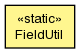## org.modeshape.search.lucene Class FieldUtil

```java.lang.Objectorg.modeshape.search.lucene.FieldUtil
```

`public class FieldUtilextends Object`Utility for working with Lucene field values.

Method Summary
`static String` `decimalToString(BigDecimal value)`
Creates a canonical string representation of the supplied `BigDecimal` value, whereby all string representations are lexicographically sortable.
`protected static String` `negate(String value)`
Compute the "negated" string, which replaces the digits (0 becomes 9, 1 becomes 8, ... and 9 becomes 0).
`protected static StringBuilder` `negate(StringBuilder value)`
Compute the "negated" string, which replaces the digits (0 becomes 9, 1 becomes 8, ... and 9 becomes 0).
`protected static void` `removeTralingZeros(StringBuilder sb)`
Utility to remove the trailing 0's.
`static BigDecimal` `stringToDecimal(String value)`
Converts the canonical string representation of a `BigDecimal` value into the object form.

Methods inherited from class java.lang.Object
`clone, equals, finalize, getClass, hashCode, notify, notifyAll, toString, wait, wait, wait`

Method Detail

### decimalToString

`public static String decimalToString(BigDecimal value)`
Creates a canonical string representation of the supplied `BigDecimal` value, whereby all string representations are lexicographically sortable. This makes it possible to store the wide range of values that can be represented by BigDecimal, while still enabling sorting and range queries.

This canonical form represents all decimal values using a prescribed format, which is based upon Steven Rowe's suggestion but with modifications to handle variable-length exponents (per his suggestion in the last sentence), use spaces between fields on where required (for minimal length), and utilize an optimized (e.g., shorter) form when the value is '0' or the exponent is '0'. Thus, this format contains only digits (e.g., '0'..'9') and the '-' character.

```     <significand-sign><exponent-sign><exponent-length> <exponent><significand>
```
where:
• the significand is the part of the number containing the significant figures, and is a (big) integer value obtained from the BigDecimal using `BigDecimal.unscaledValue()`;
• the exponent is the integer used to define the number of factors of 10 that are applied to the significand, obtained by computing `value.precision() - value.scale() - 1`;
Thus the fields are defined as:
• the `<significand-sign>` is '-' if the significand is negative, '0' if equal to zero, or '1' if positive;
• the `<exponent-sign>` is '-' if the exponent is negative, '0' if equal to zero, or '1' if positive; if '0', then the `<exponent-length>` and `<exponent>` fields are not written;
• the `<exponent-length>` is the postive value representing the length of the `<exponent>`, and is not included when the `<exponent-sign>` is '0';
• the `<exponent>` is the integer used to define the number of factors of 10 that are applied to the significand, obtained by computing `value.precision() - value.scale() - 1`;
• the `<significand>` is the part of the number containing the significant figures, and is a (big) integer value obtained from the BigDecimal using `BigDecimal.unscaledValue()`;
In the case of a negative significand, the `<significand>` field is negated such that each digit is replaced with `(base - digit - 1)` and appended by 'A' (which is greater than all other digits) to ensure that significands with greater precision are ordered before those that share significand prefixes but have lesser precision.

Thus, the format for a negative BigDecimal value becomes:

```     -<reversed-exponent-sign><negated-exponent-length> <negated-exponent><significand><sentinel>
```
where the `<sentinel>` is always 'A'. Note that the exponent length field is also negated.

### Examples

Here are several examples that show BigDecimal values and their corresponding canonical string representation:

```    +5.E-3     => 1-1 65
+1.E-2     => 1-1 71
+1.0E-2    => 1-1 71
+1.0000E-2 => 1-1 71
+1.1E-2    => 1-1 711
+1.11E-2   => 1-1 7111
+1.2E-2    => 1-1 712
+5.E-2     => 1-1 75
+7.3E+2    => 111 273
+7.4E+2    => 111 274
+7.45E+2   => 111 2745
+8.7654E+3 => 111 387654
```
Here is how a BigDecimal value of `zero` is represented:
```     0.0E0     => 0
```
BigDecimal values with an exponent of '0' are represented as follows:
```    +1.2E0     => 1012
-1.2E0     => -087A
```
And here are some negative value examples:
```    -8.7654E+3 => --8 612345A
-7.45E+2   => --8 7254A
-7.4E+2    => --8 725A
-7.3E+2    => --8 726A
-5.E-2     => -18 24A
-1.2E-2    => -18 287A
-1.11E-2   => -18 2888A
-1.1E-2    => -18 288A
-1.0000E-2 => -18 28A
-1.0E-2    => -18 28A
-1.E-2     => -18 28A
-5.E-3     => -18 34A
-5.E-4     => -18 44A
```

This canonical form is valid for all values of `BigDecimal`.

Parameters:
`value` - the value to be converted into its canonical form; may not be null
Returns:
the canonical string representation; never null or empty
`stringToDecimal(String)`

### stringToDecimal

`public static BigDecimal stringToDecimal(String value)`
Converts the canonical string representation of a `BigDecimal` value into the object form.

See `decimalToString(BigDecimal)` to documentation of the canonical form.

Parameters:
`value` - the canonical string representation; may not be null or empty
Returns:
the BigDecimal representation; never null
`decimalToString(BigDecimal)`

### negate

`protected static String negate(String value)`
Compute the "negated" string, which replaces the digits (0 becomes 9, 1 becomes 8, ... and 9 becomes 0).

Parameters:
`value` - the input string; may not be null
Returns:
the negated string; never null
`negate(StringBuilder)`

### negate

`protected static StringBuilder negate(StringBuilder value)`
Compute the "negated" string, which replaces the digits (0 becomes 9, 1 becomes 8, ... and 9 becomes 0).

Parameters:
`value` - the input string; may not be null
Returns:
the negated string; never null
`negate(String)`
`protected static void removeTralingZeros(StringBuilder sb)`
`sb` - the input string builder; may not be null